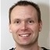0

# f´: Derivative - example??

ksilovich 6 years ago in IQANdesign updated by Gustav Widén (System support) 6 years ago

By any chance are there any examples of using the derivative function?

I am trying to measure the gallons per min of a water pump we have. In the pump there is a linear (0.5v to 4.5v) sensor. So I am trying to use the derivative function to calculate the in/sec at which the sensor is traveling to calculate the GPM. In addition to just reading the in/sec I need to know in which direction the sensor is moving, because the amount of water is different on the return stroke due to the rod diameter.

I am currently using the derivative, but the issue I am facing is that when I am reading the sensor and applying the derivative the value is very finicky (jumps around alot) and if I apply any sort of filter to the sensor input the calculated flow lags the actual flow too much for our application.is the cycletime of the MD4 10ms? Maybe it's beter to use an rpm sensor instead. Rpm * total volume of rod side and the other side when you have 2 pistons.20ms is the fastest I can go with this system. There is no spinning parts of this pump to measure RPM. The pump is rodder pump (acts just like a Hyd cylinder). After a lot of testing I think I'm to a point where I have usable speed of the rod. I used a moving average of 3 on the sensor and then filtered the data slightly to get to a point where I get in/sec with less then a half second lag time. Better then the 2 second lag time I had before.I agree, applying a moving average filter before taking the derivative of the signal is a good way of making it less noisy.